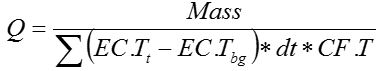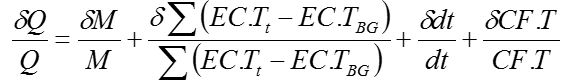# Introduction

Salt-Dilution Instream Q (SDIQ) a method in which a known mass of salt is injected upstream of a conductivity probe and the conductivity signal measured.  The technique has been used for decades.  An introduction to the technique is described here and instructions on using the technique with QiQuac are here.

# Uncertainty vs Error

Uncertainty is what we know we don’t know.  Error is the difference between our estimate of a quantity and the true value of the quantity.  The problem with the latter is that it is often difficult to measure the true value of the quantity, since all measurements of natural quantities contain error.
To get around this problem in this study, we built a model of the salt-dilution measurements where we know absolutely the true value of the quantity.  The model is described and discussed further in this post.
There are two types of Uncertainty as discussed in Assessing Uncertainty in Direct Discharge Measurements.  Type A evaluation of standard uncertainty is obtained from the statistical analysis of a series of observations determined from the current measurements.  Type B evaluations are founded on other means (prior information, expert knowledge, engineering  judgment).  An example of Type B uncertainty in our case is the difference between two independent SD IQs, or the difference between an SDIQ and a current metering IQ.

# Sources of Uncertainty

We want to identify the sources of error/uncertainty and control for them.  We start with the equation for calculating the flow in Salt Dilution(eq 1)

and find the partial derivative wrt each variable.(eq 2)

From Eq 2, only the error in CF.T and the error in the Area under the pulse (EC.Tt – EC.TBG) are significant.  The others can easily be reduced to less than 1%.   The one component not included in this estimate of error is the mixing error.  All Tracer Dilution methods assume that the tracer has been mixed across the channel, or completely mixed. See the discussion on mixing length for a more detailed examination of the topic.
Table 1 shows the significant sources of error and an estimate of the potential error introduced.  From the Summary row, we see that while there is the danger of very large error in the resulting measurement, there is also the potential for very low error if the sources of error are controlled for.  For a more detailed examination of the sources of uncertainty, see this post.

# Dosing

The introduction of NaCl into natural streams is relatively safe, so long as concentrations are kept below dangerous levels for the most sensitive species. This can be done in two ways:

1. use a low dosage (kg of NaCl per m³/s of flow) and/or
2. inject the NaCl very slowly.

The purpose of the present study is to examine the relationship between error (uncertainty) and dosing.
Table 2 is taken from Moore (2005) and shows the various dosage recommendation from the existing literature. We can see from this table that dosage recommendations vary widely between 0.2 to 2 kg/(m³/s) of flow.  The reason for the larger recommendation is in order to increase the area under the pulse.  However, from Eq 2, the error due to this component is both the magnitude of the pulse, but also the relative error in the background conductivity.  The error in the background conductivity can further be reduce to 2 components:

1. the noise in the signal and
2. the quantization error (QE).

The first should be Gaussian in nature and can be reduced to near zero if enough samples are taken.  Of course, this assumes that the background conductivity is stable over the course of the measurement, which we’ve found not to be the case, as discussed in the Introduction to SD.  The second source of error is the quantization error and typically taken as ½ the resolution of the sensor.  This simply means that while the true value is between quanta in the digital signal, we do not know where between the adjacent quanta the true value is.  If large enough random error is layered on top of the quantization error, then by taking an average over time, we can arrive at a close approximation of the true value simply from the frequency of the two adjacent quanta.  For most instruments, this is not the case, however, since the resolution and the stated accuracy of the instrument are closely related and manufacturers are hesitant to provide a resolution much finer than the accuracy of the device.  This is a handicap of most instruments, we feel, since the accuracy of a SD measurement is a function of our ability to estimate the BG EC.T as close as possible, thereby eliminating the quantization error.  The goal of the T-HRECS device was to eliminate the potential quantization error.  Therefore, the signal may look noisy, but that is better than a static value.

We recognize that the quality of the SD measurement, and the magnitude of the uncertainty, is actually a function of the SNR. The noise in the SNR is whichever is larger of the standard error in the EC.TBG or ½ the QE.  For instrument resolution of 0.1 μS/cm and low BG noise, a rise of 20 μS/cm peak over background is, on average, an average Signal above BG of 2.0 μS/cm. The ratio of 2.0 μS/cm to 0.1 μS/cm results in a SNR of 20.  If we take the reciprocal of the SNR and consider this to be the EC.T error, this introduces 5% uncertainty into IQ measurement.  A rise of 50 μS/cm peak over background is, on average, an average Signal above BG of 5.0 μS/cm.  The ratio of 5.0 μS/cm to 0.1 μS/cm results in a SNR of 50.  If we take the reciprocal of the SNR and consider this to be the EC.T error, this introduces 2% uncertainty into IQ measurement.  If the sensor resolution is 0.01 μS/cm, then we can use 10x less salt to achieve the same SNRs as the sensor with resolution 0.1 μS/cm.  Again, this is why T-HRECS has a resolution of 00.01 up to 99.99 μS/cm and 100.0 to 999.9 μS/cm.
Part of the reason for this SNR-based approach is to avoid overdosing.  This can be the case when the user is attempting to increase the EC.T by some factor over the BG EC.T. THIS SHOULD NOT BE DONE! This is both a hazard to aquatic habitat and species, and a waste of salt.  Know your sensor, and know the background noise, and inject accordingly.
It should also be noted that since we are working primarily in EC.T, the temperature compensation becomes a component of the SNR.  The temperature accuracy and noise is layered on top of the EC noise.  Hopefully, the change in temperature over the course of the measurement is insignificant, but this cannot be assumed.  We’ve made measurements at inland streams during freshet where the water temperature has changed by up to 1˚C over the course of the measurement.  This is also a function of the duration of the measurement.  The duration of the measurement is a function of both the mixing length and the average velocity of the stream.  For very low flows, or where there are pools, or very long-mixing lengths, the time for the pulse to pass can exceed 1 hour.  It is a function of the flow:storage ratio.  If the entire mixing length is considered to be storage, the transit time is that time required for all of the salt to leave the storage.  This is not simply the total storage divided by the flow rate since the concentration decreases over time and approaches infinity as the last particle of NaCl may never leave the storage volume.  There is no need to worry about such existential questions so long as the practitioner realizes that the more storage there is relative to the flow rate, the longer the transit time.

# Results

Figure 1 shows the results of analysis of 343 SDIQs, comparing the Peak over BG EC.T as a function of dosage (kg/(m³/s)). We can see in this figure that the shorter transit time pulses, categorized in the 10 min bin, have a higher Peak over BG EC.T than a similar dosage for a longer transit time. Likewise, the longest transit time injections have a much lower Peak over BG EC.T than similar dosages for shorter transit times. Also note that the peaks are all below 50 μS/cm.

We propagated the total uncertainty through equation 2 for all of these measurements and the results are shown in FIG 2. The points were again grouped, this time into EC.T Uncertainty which is a function of both the sensor resolution and the background noise.  This figure shows the lowest uncertainty occurs when EC.T Unc. is smallest.  There is a baseline of ~3% uncertainty stemming from CF.T and NaCl Mass uncertainty.

When the uncertainty due to CF.T and Mass is removed, we can see the uncertainty due to the SNR alone in Figure 3.  It’s interesting to note that the uncertainty is mostly below 5% until the dosage drops below 0.7 kg/m³/s.

We can further explain variance by transit time of pulse, shown in Figure 4.  The faster the transit time, assuming completely mixed, the lower the uncertainty. 0.1 µS/cm is typical of many commercial sensors for EC.T < 200 µS/cm.  This figure suggests that for a sensor with 0.1 µS/cm resolution, 1 kg/(m³/s) should be used, unless the transit time is 10 mins or less, then 0.5 kg/(m³/s) could be used.  We’ve defined a Target Range in this figure of less than 5% uncertainty and remaining below a dosage of 1 kg/(m³/s).Figure 4: %Uncertainty vs Dosage as a Function of Transit Time for EC.T Unc. = 0.1 uS/cm

Shown in Figure 5, if we can attain EC.T Unc. of 0.02 µS/cm (requires finer than 0.02 µS/cm resolution), then we can reduce the dose to 0.2 kg/cms and remain below 5% Uncertainty.Figure 5: %Uncertainty vs Dosage as a Function of Transit Time for EC.T Unc. = 0.02 uS/cm

EC.T Unc. of 1 µS/cm or greater is shown in Figure 6.  The EC.T Unc. is the combined sensor resolution, background noise, and return to background EC.T.  Only two measurements are in the target range. It is possible to get high quality measurement, but this requires a larger dose.Figure 6: %Uncertainty vs Dosage as a Function of Transit Time for EC.T Unc. = 1.0 uS/cm

# Conclusions

The relationship shown in previous figures is tabulated in Table 3 for two data classes, Uncertainty less than 5% and less than 10%.  The NaCl dose depends on EC.T Unc. and transit time.  Most common EC.T Unc. and transit time (0.1 uS/cm and 30 mins) requires 1.1 kg/(m³/s) to attain ±5% at 95%confidence.  A shorter transit time or lower EC.T Unc. results in lower required dose.

We’d like to propose a new Rule of Thumb for dosing in Salt Dilution Studies

• Use 10x the EC.T Unc. at 30 mins.
• Half the dose for 10 mins, double for 60 mins.
• Use a maximum of 2 kg/cms.

and to reduce the concentration at the point of injection, we suggest a

• Best Practice: Pre-dissolve or inject slowly (>30 second).

# References

Moore, R.D. (2005)“Slug Injection Using Salt in Solution” Streamline Watershed Management Bulletin Vol. 8/No. 2 Spring 2005, Victoria, B.C.